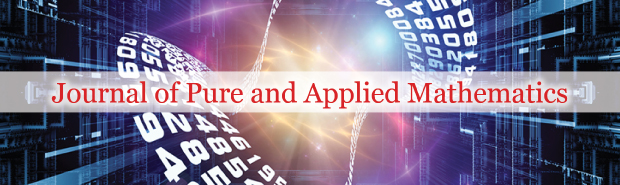All submissions of the EM system will be redirected to Online Manuscript Submission System. Authors are requested to submit articles directly to Online Manuscript Submission System of respective journal.### Abundant M-Fractional Exact Solutionsfor STOand (3 + 1)-Dimensional KdV-ZK Equations via (m + (G\'/G))-Expansion Method

Author(s): Sharafat Ali

In the field of applied mathematics, fractional calculus is used to contract with derivative as well as the integration of any power. Different definitions of the fractional derivative have been introduced in the literature. For example, these are some important definitions of fractional derivatives, Riemann-Liouville derivative, Caputo derivative and conformable derivative. Recently the generalization of the conformable derivative has been given as M-fractional conformable. Fractional differential equations (FDEs) “equations involving fractional derivatives” are employed invarious areas of science and engineering and others [1-5] have widely been interested. That’s why they have gained many attractions from many researchers. To acquisition, the analytical solutions of the FPDEs is a conspicuous look of scientific research.

PDF